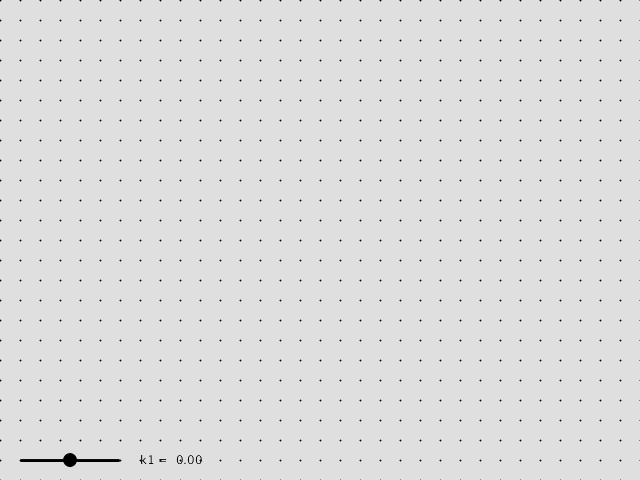# 最小二乘问题（十四）

“可是上一篇中我们不是已经有了一个新的并且没有 $\Delta x_1$ 的标准方程了吗？”是这样没错，但回忆上一篇，我们得到的那个问题中，$x_1$ 变量被固定了，也就是说并不是 $\Delta x_1$ 被忽略了，而是它被固定为了 $\Delta x_1 = 0$ 。而这里我们所希望的是让它可以随心所欲，因此我们需要用另外一个技巧：高斯消元。

# 径向畸变的效果Explanation: Black dots should form a rectangular grid. However, if the camera lens has radial distortion, image will be distorted. This animation simulates the photo under different radial distortion parameters. More specifically, the distorted image point is given by $p \mapsto L(r)\times p$, where $L(r) = 1+k_1\times r$, and $r = \|p-c\|$.

# 深藏功与名

float bb_min, bb_max;
for(int i = 0; i < 3; ++i) {
bb_min[i] = std::numeric_limits<float>::max();
bb_max[i] = std::numeric_limits<float>::min(); // min 给出的是最小的绝对值
}
for(int j = 0; j < model.vertex_num(); ++j) {
for(int i=0;i<3;++i) {
bb_min[i] = std::min(bb_min[i], model.vertex(j)[i]);
bb_max[i] = std::max(bb_max[i], model.vertex(j)[i]);
}
}